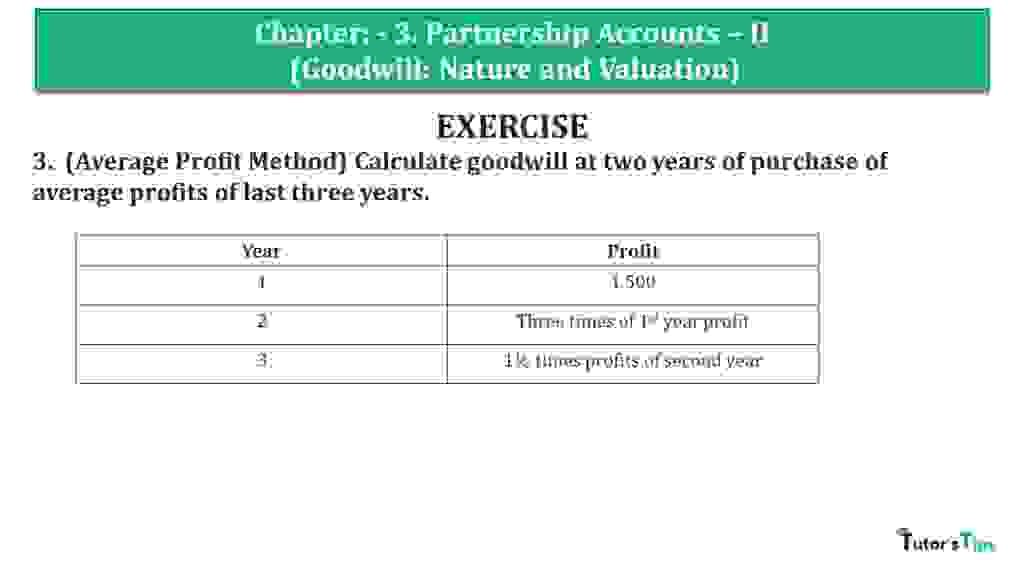# Question 03 Chapter 3 of +2 Part-1 – USHA Publication 12 Class Part – 1Question 03 Chapter 3 of +2-Part-1

3. (Average Profit Method) Calculate goodwill at two years of purchase of average profits of last three years.

 Year Profit 1 1,500 2 Three times of 1st year profit 3 1½ times profits of second year

### The solution of Question 03 Chapter 3 of +2 Part-1: –

 Average Profit = Total Profit for past given years Number of years = 1,500 + 4,500 + 6,750 3 = 12,750 3 = 4,250

 Number of years’ purchase = 2 Goodwill = Average Profit X Number of years’ purchase Goodwill = 4,250 X 2 Goodwill = 8,500

Working Note : –

*1 Calculation of Profits of last three years

 1st year’s Profit = 1,500 2nd year’s Profit = Three times of 1st year profit = 1,500 X 3 = 4,500/-

 1st year’s Profit = 1,500 2nd year’s Profit = 1½ times profits of second year = 4,500 X 1.5 = 6,750/-